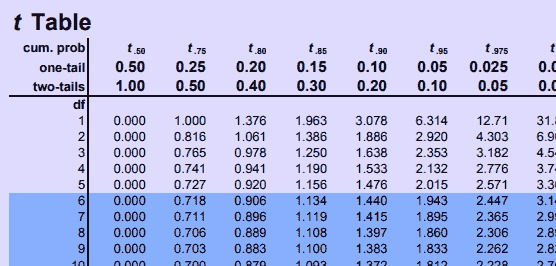top of page

# T-Distribution## Use the T-distribution when:

• Your sample size is small (less than 30)

• and/or your population standard deviation σ is unknown

The law of large numbers tells us that the larger your sample size, the more accurate your results will be.

Small sample sizes can be a problem, but sometimes that's all we have.

So the solution is to use the T-Distribution.

The T-Distribution (T-Table) takes into account the size of your sample when determining probability by using degrees of freedom.

The equation for calculating a t-score is very similar to that for a z-score, except we include the sample standard deviation and sample size rather than the population standard deviation:### Example

Suppose we take a sample of 5 test scores from a population of all students in the nation.

The national test score average is believed to be a score of 7.

Our sample of 5 students has a mean score of 7.8 and standard deviation of 1.72.

We want to know if the national average is actually higher than 7.

To do this we can test our sample average to see if it is significantly higher than the national average. In other words, we'll see if it is probable that most students will score higher than 7 based on the results of our sample.

Hypothesis: Same

Hypothesis: Different (greater, less, not equal to)

Hypothesis always refers to the population mean.

H-null: p = 7 Is the mean equal to 7

H-alternative: p > 7 Is the mean higher than 7

We are going to test the alternative hypothesis using the t-score and t-distribution.

We can plug into the formula:Finding the Probability

Now we know that our sample mean (7.8) is 1.04 standard deviations above the population mean in our example (7).

We can can use the T-Distribution find the probability of achieving a score that is 1.04 standard deviations above the mean.

If it is likely to achieve such a high score, then the difference is not significant.

However, if it is unlikely to achieve such a high score, then it is significant. We need to rethink our null hypothesis that the population mean score is 7 - as an unlikely event has occurred.

Use the T* Critical value - Visual Examples

- How to read the Student's T Distribution Table

- Significance Level (alpha)

- Degrees of freed (n-1)

- One vs. Two tails

Use T Table to Estimate P-Value

Use a Calculator

In this case, the likelihood of scoring 7.8 is probable (higher than .05). Which suggests that this mean is not significantly different than the population. So, the hypothesized population mean of 7.0 can remain.

Opposite example - In this case, we can reject our null hypothesis that the true mean of the population is 7. Our sample suggests that the true mean is actually higher.

Example Left Tailed

Example 2 Tailed

## Calculators

The easiest way to calculate a t score, and find it's probability (p-value) is to use a statistics calculator.

Graphing calculators, statistical software programs (such as Excel, SPSS, R), or free online calculators are all able to T-Tests, which give you the t-score and resulting probability for a value in a dataset.